# Volume of Cone

Go back to  'Mensuration-Solids'

It was easy to determine the expressions for the volumes of a cuboid and a cylinder with much rigorous analysis. However, the expression for the volume of a cone is not that straightforward to obtain. This is because the cross-sectional area of the cone is changing as we move along its height. Hence, we cannot use the relation Base Area × Height to obtain a cone’s volume.

We will use a more involved technique to evaluate the volume of a cone. The discussion which follows might be slightly challenging, so you are urge to read it multiple times until you have complete clarity.

As we said earlier, the problem with using Base Area × Height for a cone is that the cross-sectional area of the cone changes as we move along its height. However, suppose that we divide the cone into a large number of slices. Now, observe any one slice: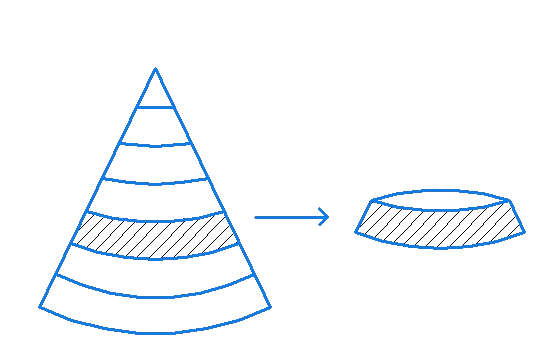Can we calculate the volume of this slice? Note that even this slice is not a right circular cylinder, since the curved edges of the slice are not perpendicular to the base. However, since the thickness of the slice is very small, we can approximate the slice as a cylinder: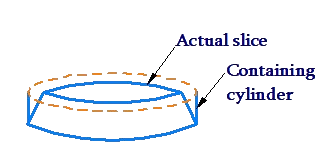If the radius of the lower base of the slice is r and its thickness is h, we can approximate the slice as a right circular cylinder of base radius r and height h. This is possible because the difference between the volumes of the slice and the cylinder will be small, as the figure above shows (the smaller the thickness of the slice, the smaller will this difference be).

Having understood this, think about how we can calculate the volume of the cone (take its radius to be R and height to be H). Simple! We cut up the cone into a (large) number of such slices, measure the volume of each slice, and then add up all these volumes. The larger the number of slices, the more accurate our answer will be (because for each slice, our calculated volume will be closer to the actual volume).

Suppose that we divide the cone into N slices, where N is some large number. Number the slices from 1 to N, starting from the lowermost slice (the topmost slice is actually a cone):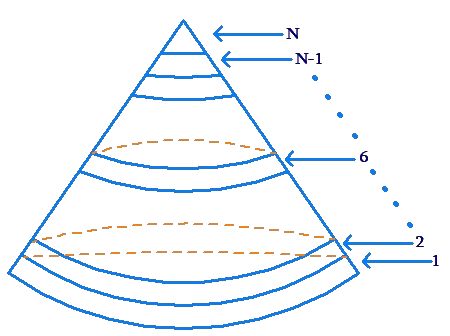Focus your attention on the ith slice. What is the radius of (the base of) this slice? What is its thickness? To determine the thickness is simple. The height of the cone is H, and we have divided it into N slices of equal thickness. Thus, the thickness of each slide is $$d = \frac{H}{N}$$. To calculate its radius r, note that the ith slice has $$\left( {i - 1} \right)$$ slices below it, with each slice being of thickness d. Thus, the height of the ith slice above the base is $$\left( {i - 1} \right)d$$, as the following figure shows: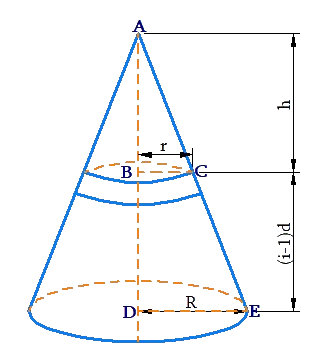We note that  $$\Delta ABC \sim \ \Delta ADE$$ , so that

\begin{align}& \frac{{AB}}{{AD}} = \frac{{BC}}{{DE}}\\& \Rightarrow \,\,\,\frac{h}{H} = \frac{r}{R}\\& \Rightarrow \,\,\,r = \frac{{hR}}{H} = \frac{{\left( {H - \left( {i - 1} \right)d} \right)R}}{H}\\& \qquad\qquad \qquad = \left( {1 - \left( {i - 1} \right)\frac{d}{H}} \right)R\end{align}

Since $$d = \frac{H}{N}$$, we have $$\frac{d}{H} = \frac{1}{N}$$, and so:

$r = \left( {1 - \frac{{\left( {i - 1} \right)}}{N}} \right)R$

Now, we have both the radius (of the base) and the thickness of the ith slice. Thus, we can approximate its volume as that of a cylinder with the same radius and height: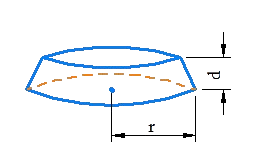Thus,

${V_i} \approx \pi {r^2}d = \pi {\left( {1 - \frac{{\left( {i - 1} \right)}}{N}} \right)^2}{R^2}d$

Using $$d = \frac{H}{N}$$, this becomes

\begin{align}&{V_i} \approx \pi {\left( {1 - \frac{{\left( {i - 1} \right)}}{N}} \right)^2}\frac{{{R^2}H}}{N}\\&\,\,\,\,\, = \frac{{\pi {R^2}H}}{{{N^3}}}{\left( {N - \left( {i - 1} \right)} \right)^2}\end{align}

This expression gives us the volume of the ith slice in terms of i (and the other parameters which are all constants).

To calculate the volume of the cone, we add up all these slice-volumes:

${V_{cone}} = {V_1} + {V_2} + {V_3} + ... + {V_n}$

In each term on the right, the expression $$\frac{{\pi {R^2}H}}{{{N^3}}}$$ is common. Only the term inside the bracket is changing (because the parameter i is changing). Thus,

\begin{align}&{V_{cone}} = {V_1} + {V_2} + {V_3} + ... + {V_n}\\ &\qquad= \frac{{\pi {R^2}H}}{{{N^3}}}\left( {{N^2} + {{\left( {N - 1} \right)}^2} + ... + {1^2}} \right)\end{align}

We are very near to our answer now! The expression inside the bracket is the sum of the squares of the first N natural numbers. The value of this sum is

$\frac{{N\left( {N + 1} \right)\left( {2N + 1} \right)}}{6}$

This can be proved by a number of techniques into which we will not go at this point. Thus, the volume of the cone is

\begin{align}&{V_{cone}} = \frac{{\pi {R^2}H}}{{{N^3}}}\left\{ {\frac{{N\left( {N + 1} \right)\left( {2N + 1} \right)}}{6}} \right\}\\&\,\,\,\,\,\,\,\,\,\, = \frac{{\pi {R^2}H}}{6}\left\{ {\frac{{N\left( {N + 1} \right)\left( {2N + 1} \right)}}{{{N^3}}}} \right\}\\&\,\,\,\,\,\,\,\,\,\, = \frac{{\pi {R^2}H}}{6}\left\{ {\left( {1 + \frac{1}{N}} \right)\left( {2 + \frac{1}{N}} \right)} \right\}\end{align}

Now, for the final step, which also happens to be really important! Remember how we talked about approximating the volume of any slice using the volume of a cylinder of the same dimensions. The approximation will get better and better as the thickness of the slice reduces.

This means that if we cut up the cone into a really large number of slices (if N is really large), and add up the volumes of these slices, we will obtain a value very close to the actual value of the cone.

If N goes to infinity, we will obtain the exact volume of the cone. However, if $$N \to \infty$$, then $$\frac{1}{N} \to 0$$ (this simple means that if N is really large, then $$\frac{1}{N}$$ is really small – close to 0). Thus, the exact volume of the cone can be calculated by substituting $$\frac{1}{N} \to 0$$ in the expression above:

\begin{align}&{V_{cone}} = \frac{{\pi {R^2}H}}{6}\left\{ {\left( {1 + \frac{1}{N}} \right)\left( {2 + \frac{1}{N}} \right)} \right\}\\& \qquad\qquad\qquad\;\; \left( {{\rm{where }}\frac{1}{N} \to 0} \right)\\&\,\,\,\,\,\,\,\,\,\, = \frac{{\pi {R^2}H}}{6}\left\{ {\left( {1 + 0} \right)\left( {2 + 0} \right)} \right\}\\&\,\,\,\,\,\,\,\,\,\, = \frac{{\pi {R^2}H}}{6} \times 2\\ &\Rightarrow \,\,\,{V_{cone}} = \frac{1}{3}\pi {R^2}H\end{align}

Thus, if the cone’s base radius is r and height is h, its volume is given by

$V = \frac{1}{3}\pi {r^2}h$

A cone’s volume is one-thirds of the volume of the right circular cylinder with the same base and height: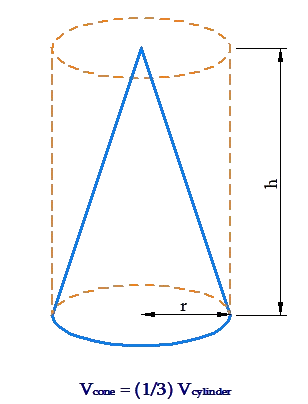Example 1: Find the total surface area and volume of a cone whose base radius is 3 cm and height is 5 cm.

Solution: The slant height of the cone is:

$l = \sqrt {{r^2} + {h^2}} = \sqrt {{3^2} + {5^2}} = \sqrt {34}cm$

We have the total surface area as:

$TSA = \pi rl = \pi \left( 3 \right)\left( {\sqrt {34} } \right) \approx 54.9\,{\rm{c}}{{\rm{m}}^2}$

The volume will be

\begin{align}&TSA = \pi rl = \pi \left( 3 \right)\left( {\sqrt {34} } \right) \approx 54.9\,{\rm{c}}{{\rm{m}}^2}\\&V = \frac{1}{3}\pi {r^2}h = \frac{1}{3}\pi {\left( 3 \right)^2}\left( 5 \right) \approx 47.1\,{\rm{c}}{{\rm{m}}^3}\end{align}

Example 2: A conical building has a base radius of  50 m and a height of 40 m. The building is vertically divided into two halves: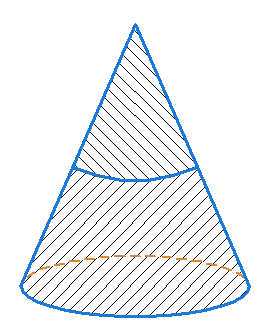The bottom half (according to height) of the building needs to be colored with a paint which costs INR 150 / m2. The top half needs to be colored with a paint which costs INR 300 / m2.

(a)   Find the cost of this paint job.

(b)   What is the ratio of the volume of the bottom half to that of the top half?

Solution: (a) The total curved surface area of the building is

\begin{align}&{S_1} = \pi rl = \pi r\sqrt {{r^2} + {h^2}} \\&\,\,\,\,\,\, = \pi \left( {50} \right)\sqrt {{{50}^2} + {{40}^2}} \approx 10,053\,{m^2}\end{align}

The radius of the top half of the building will be half that of the base radius (why?). Thus, the curved surface area of the top half is

${S_2} = \pi \left( {25} \right)\sqrt {{{25}^2} + {{20}^2}} \approx 2,513\,{m^2}$

This means that the curved surface area of the bottom half is

${S_3} = {S_1} - {S_2} \approx 7540\,{m^2}$

The cost of the paint job will be

\begin{align}&{S_3} \times 150 + {S_2} \times 300\\ &= 7540 \times 150 + 2513 \times 300\\& \approx {\rm{INR }}\;18.85\,{\rm{lacs}}\end{align}

(b) The total volume of the building is

\begin{align}&{V_1} = \frac{1}{3}\pi {r^2}h = \frac{1}{3}\pi {\left( {50} \right)^2}\left( {40} \right)\\ &\qquad\qquad \,\,\,\,\,\,\,\,\, \approx 1,04,667\,{{\rm{m}}^3}\end{align}

The volume of the top half will be

\begin{align}&{V_2} = \frac{1}{3}\pi {\left( {25} \right)^2}\left( {20} \right)\\&\,\,\,\,\,\, \approx 13,083\,{{\rm{m}}^3}\end{align}

Thus, the volume of the bottom half will be

\begin{align}&{V_3} = {V_1} - {V_2}\\&\,\,\,\,\,\, \approx 104667 - 13083\\&\,\,\,\,\,\, = 91,584\,{{\rm{m}}^3}\end{align}

The required ratio is

$\frac{{91,584\,{{\rm{m}}^3}}}{{13,083\,{{\rm{m}}^3}}} = 7$

Mensuration and Solids
Mensuration and Solids
grade 10 | Questions Set 2
Mensuration and Solids
Mensuration and Solids
grade 10 | Questions Set 1